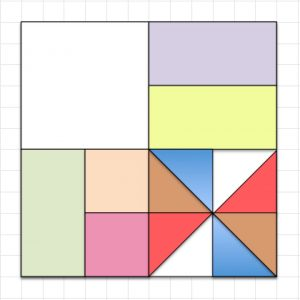The square is one unit and it is split into smaller parts. Copy the picture and label each part with the fraction it shows.

Which coloured bits would you use to show that two eighths equals one quarter? $$\frac{2}{8}=\frac{1}{4}$$

Which coloured bits would you use to show that two sixteenths equals one eighth? $$\frac{2}{16}=\frac{1}{8}$$

What can you say about the fractions shown by the triangles?

How many small pink squares give the same fraction as the three white bits taken together?

Write down fractions that add up to one half in different ways using the fractions shown in the diagram.

Draw your own pattern in a square and label the fractions in your pattern.

Tagged with: